Explore BrainMass

# The Capital Asset Pricing Model (CAPM)

Many of the concepts we use in finance involve discounting risky cash flows using a discount rate appropriate for the level of risk associated with the investment. Investors will only hold risky securities or invest in risky projects if the expected rate of return on an investment is at least as much as the discount rate. This is because all investors are risk-adverse, that is, when they take on additional risk they expect to be compensated. This maxim is fundamental for an efficient market.

Therefore, in order to value a company's stock we need to find a way to measure its risk. Typically, we use variance or standard deviation to measure the risk of an individual stock. However, in the real world, investors hold more than one stock. When investors hold a portfolio of stocks, the risk of an individual stock becomes less important - what we really want to know is how an individual stock contributes to the overall risk of the portfolio. This is because the larger a portfolio is, the more an investor can diversify away her risk. As a result, most investors would want to diversify their portfolios as much as possible. Knowing this, we can imagine that an investor would expect a return from a security based on the level of risk each security contributes to a large portfolio. If we assume that each investor holds a very large portfolio, we can use the market portfolio in our calculation for the expected return of a stock. The market portfolio is a hypothetical portfolio consisting of every stock on a market.

The capital asset pricing model tells us that an investor's expected return on a security is equal to the market's risk free rate of return (what the investor would expect to recieve as a return on a riskless investment such as a T-Bill) plus some amount of additional return the investor expects for investing in a risky stock, often called a risk premium. A stock's risk premium is equal to the risk premium of the market porfolio (expected return on the market portfolio minus the risk free rate) adjusted for or multiplied by the stock's Beta, which tells us how much risk the stock would contribute to a market porfolio. We can see from this formula that the Beta of the market portfolio itself is one, that a stock with a Beta less than one would require a smaller risk premium than the market portfolio, and a risky stock with a Beta of more than one would require a larger risk premium than the market portfolio.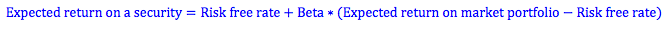Deriving The Capital Asset Pricing Model

The capital asset pricing model is derived from finding the expected return of an individual stock as well as its variance or standard deviation. Once we know a stock's variance or standard deviation, we can find its covariance or correlation with the expected return of other stocks or the market portfolio. To simplify these steps most students will be given a stock's Beta, which tells us how much the stock will move in response to movements in the market place and is a function of the stock and market's variance. By using Beta, the capital asset pricing model makes it easy for students to find the expected return of a stock if it was part of a large portfolio.

Expected Return (R̄): The rate of return that an investor expects a single stock to earn over the next period. This may be based on the average return of the stock in the past, a detailed analysis of the firm's prospects, a computer simulation, or inside information.

Variance (σ2) and Standard Deviation (σ): To find variance, we look at the deviation of a stocks actual return to its expected return. These deviations are squared, and then averaged to get the variance of a stock. Standard deviation is equal to the square root of variance. We can think of standard deviation as the standardized form of variance.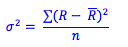Where,
R = Actual return
n = number of states or observations
R - = deviation of a stocks actual return from its expected return

Covariance (σA,B): When we find variance, we look at the deviations of a stock's actual return from its expected return. To find covariance, we look at these same deviations. However, we know what to know how they relate to the deviations of another stock. To get the covariance, we find the product of the deviations of two stocks in each state. The average of these products is the covariance.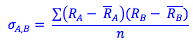Correlation (ρA,B): The correlation is the standardized covariance. It is equal to the covariance divided by the standard deviation of each individual stock. Correlation is always between +1 and -1.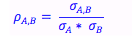Variance of a two-stock portfolio (σP2): The variance of a portfolio consists of the variances of the individual securities, the covariance of the two securities, and the percentage of the portfolio made up by each security (XA and XB). A positive covariance will increase the variance of the entire portfolio. A negative covariance will reduce the overall risk of the portfolio. Creating a portfolio with securities that have a negative covariance so that they offset each other is known as a hedge. For a two-stock portfolio, we have the following variance:

Using Covariance: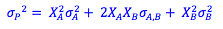Using Correlation: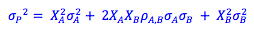We can extrapolate this to find the variance of the market portfolio. The capital asset pricing model suggests that the market portfolio is the only fund in which an investor needs to invest, along with an investment in a risk-free asset depending on the investor's tolerance of risk.

Beta: Beta measures the responsiveness of a security to movements in the market portfolio. We find beta by finding the covariance of the stock and market portfolio returns, and dividing this by the variance of the market portfolio.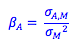Expected return of the market: If an investor wanted zero risk, he could invest in a security such as a US Treasury Bill which offers a risk-free rate of return. Because investors are risk-adverse, when they invest in risky securities, they expect to be compensated for their risk with a rate return higher than the risk-free rate. As a result, we often represent the expected return on the market in the following form, where is the expected return on the market portfolio, and RF is the risk-free rate of return.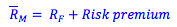Expected return on an individual security: The beta of a security reflects the risk of a security as it relates to the market portfolio as a whole. Therefore, using beta we should be able to find the expected return of an individual security if we know the expected return of the market and the risk free rate. Where ( - RF ) = Risk premium. This is the capital asset pricing model (CAPM).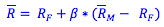There is a positive linear relationship between the beta of a security and its expected return. The market portfolio has a beta of 1, and a stock with a beta of one would have the same expected return as the expected return of the market.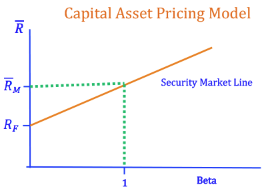Photo by Sammie Vasquez on Unsplash

© BrainMass Inc. brainmass.com October 6, 2022, 10:12 am ad1c9bdddf

## BrainMass Solutions Available for Instant Download

### CAPM and risk free return

Two mutual fund managers are being evaluated for their performance in the last ten years. One of them, Mr. Harrods, has achieved an eye-popping 34% annual average return; the other, Ms. Evans, has obtained a modest 12% annual average return. On closer examination of their portfolios, it is found that Mr. Harrods always bet on ri

### Expected return problem

Assume that you can borrow and lend at a riskless rate of 5% and that the tangency portfolio of risky assets has an expected return of 13% and a standard deviation of return of 16%. (a) What is the highest level of expected return that can be obtained if you are willing to take on a standard deviation of returns that is at mo

### Calculation of the Weighted Average Cost of Capital

Estimate your firm's Weighted Average Cost of Capital. Assume that the current risk-free rate of interest is 3.5%, the market risk premium is 5%, and the corporate tax rate is 21%. Debt: Total book value: \$10 million Total market value: \$12 million Coupon rate: 6% Yield to Maturity: 5% Common Stock: Total book value: \$1

### True false questions about CAPM

Are the following statements true or false? (a) Stocks with a beta of zero offer an expected rate of return of zero. (b) The CAPM implies that investors require a higher return to hold highly volatile securities. (c) You can construct a portfolio with a beta of 0.75 by investing 0.75 of the investment budget in bills an

### Short Term Financial Risk Concepts

Examine the concept of financial risk by answering the following questions: (a) How does the risk of a portfolio change as the number of assets in the portfolio increases? (b) Provide an example of a unique risk that can be reduced by portfolio diversification. (c) Provide an example of a market risk that cannot be reduced by po

### Finance: CAPM

1: Expected Return: Discrete Distribution The market and Stock J have the following probability distributions: Probability Rm Rj 0.27 12.25% 25.40% 0.44 6.65 10.36 0.29 21.30 36.57 (a) Calculate the expected rates of return for the market and (b) Calculate the standard deviations for the Stock J. Work with at

### Financial Ratio Analysis for Apple Inc

Review the balance sheet and income statement of Apple Inc's 2015 Annual Report. Calculate the following ratios using Microsoft® Excel®: Current Ratio Quick Ratio Debt Equity Ratio Inventory Turnover Ratio Receivables Turnover Ratio Total Assets Turnover Ratio Profit Margin (Net Margin) Ratio Return on Assets Ratio

### Capital Asset Pricing Model and Stock Valuation and Growth Rate

Using data from our fictitious Company, MT 217 (from attached sheet), we will calculate the expect value of its stock using the Constant Growth Model (attached): Po = D1/(r - g) To do that we will have to estimate the vales of r, g, and D1. To estimate the value of r we will use the Capital Asset Pricing Model: CAPM = R

### Securities and Portfolio Return Computations

Beginning Stock Price \$73 Ending Stock Price \$82 Dividend \$1.20 Percentage Total Return = #NAME? CHAPTER 10: PROBLEM 12 Stock Return the past 5 years -18.35% 14.72% 28.47% 6.48% 16.81% Holding Period Return for the Stock = #NAME? (Note: Subtract your answer by 1 to

### CAPM Analysis

Briefly set out arguments in favour of - and against - the Capital Asset Pricing Model (CAPM), outline its uses and make a critique of its underlying assumptions.

### Hedge Fund Return Analysis

1) What is the average annual (historic) return on the hedge fund? Note the returns are reported monthly. Multiply by 12 to annual returns. Need to answer with annual returns. Answer should go to 1 place behind decimal (ie: 10% expressed as 10.0) 2) What is the average (historic) return on the stock market? Note the re

### CAPM portfoio

Download the most recent 5 years of monthly data for VTI, Proctor and Gamble (PG), Exxon Mobil (XOM), Apple (AAPL), Alcoa (AA), Century Aluminum (CENX) and the 3-month T-Bill (^IRX) using Yahoo Finance. VTI is a low-cost ETF that tracks the Wilshire 5000 index and is our proxy for the market return. For the stocks and the ET

### CAPM and the Constant Dividend Growth Model

Capital Asset Pricing Model The Capital Asset Pricing Model (CAPM) is a powerful analytical tool used for calculating the price of common stock. After reflecting on theory and application of the CAPM model and reviewing the prior work on the Constant Dividend Growth Model post a one paragraph response to the following questions

### Calculation of Required Rate of Return and Asset Beta

Please help with the following problems. Provide step by step calculations for each. Acme currently has a capital structure of 20% debt to total assets, based on current market values. The current debt is riskless and more debt can be taken on, up to a limit of 35% debt, without making the debt risky and losing the firm's ab

### The Efficiency of the Market Portfolio

Question 1 The Debt Cost of Capital 14. In mid-2012, Ralston Purina had AA-rated, 10-year bonds outstanding with a yield to maturity of 2.05%. a. What is the highest expected return these bonds could have? b. At the time, similar maturity Treasuries have a yield of 1.5%. Could these bonds actually have an expected return e

### DCF Method, CAPM Method, Investment Projects

Please help with determining ratios. The attached spread sheet is FEDEX 3 year financial statements. Trying to figure out the ratios. The one that I figured out is not coming close to what Morning Star: http://financials.morningstar.com/ratios/r.html?t=FDX&region=usa&culture=en-US or MSN Money:http://investing.money.msn.com/inve

### Finance Problems: Required Rate of Return

2. Required Rate of Return AA Industries stock has a beta of 0.8. The risk-free rate is 4% and the expected return on the market is 12%. What is the required rate of return on AA's stock? 10. Portfolio Required Return Suppose you manage a \$4 million fund that consists of four stocks with the following investments: Stock

### Explaining Cost of Capital, Risk & Return, Hurdle Rate, Cost Structure, Depreciation, Call Options

Please include in-text citations and references used. Thank you. 1. Cost of Capital - If you were going to start a company, let's say a restaurant, and I was going to take \$400,000 to get it opened, how would you finance the initial investment? Things to consider are debt, equity, terms, and sources. 2. Risk & Return - G

### Portfolio Optimization Questions

1. Evaluate whether the following statements are true or false. a) Even if a risky security has a return lower than the risk-free rate, this security could be held for diversification purposes. b) The Glass-Steagall Act of 1933 separated commercial banking from investment banking. c) If returns on two stocks are perfectly p

### CAPM, SML, and Investors

The Capital Asset Pricing Model (CAPM) is a widely used concept in finance. The model is expressed graphically by the Security Market Line (SML). Within the context of investment, explain how CAPM can be useful to investors.

### Capital Asset Pricing Model Questions

Consider the following information: Stock A Stock B T-bills Beta 0.6 1.2 0.0 Expected return, % 5.0 8.0 2.0 (a) Assuming that all stocks are priced correctly according to the CAPM, compute the expected return on the market portfolio. (c) Is it possible for

### The Capital Asset Pricing Model & CAPM

In one page explain what you think is the main 'message' of the Capital Asset Pricing Model to corporations and what is the main message of the CAPM to investors?

### Corporate Finance and Pricing Models

I need 100 word original notes in answering the following questions: 1. What is operating leverage and how does it influence a project? 2. What are the two methods for estimating debit cost of capital, and what do you do when there is default risk? Explain the circumstances in which you would use each method. 3. In what

### Cost of Equity for Google

1. Show the work you did to obtain the cost of equity for Google.. 2. Is this cost of equity higher or lower than you expected? The average cost of capital for a firm in the S&P 500 is 8.2 percent. Would you think your firm should have a lower or a higher cost of capital than the average firm? 3. Look up the betas for some of

### Calculating cost of equity using capital asset pricing model

Current Yield to Maturity (YTM) on a U.S. Government bond that matures based on the Treasury Bill Rate for 1 year is 0.10 and for 13 weeks is 0.02. For Amazon.com the following is assumed: Beta 0.77 RF 5% RF = 1 RM =5 RM - RF= 4 What is the cost of equity for Amazon.com? Based on the Betas of Ebay and Overstock.com com

### Computation of rate of returns for Procter & Gamble

I need assistance with the following assignment: Estimating the cost of equity or the rate of return that Procter and Gamble's shareholders 'require'. The CAPM states the following equilibrium relationship between the (excess) rate of return that shareholders of a particular company "j" require (or actually in some sense 'de

### Which of the following projects should the firm accept?

2. Bloom and Co. has no debt or preferred stockit uses only equity capital, and has two equally- sized divisions. Division X's cost of capital is 10.0%, Division Y's cost is 14.0%, and the corporate (composite) WACC is 12.0%. All of Division X's projects are equally risky, as are all of Division Y's projects. However, the pr

### Evaluation of Analysis Measures

For an organization owning multiple assets where their core business is not real estate is CAPM recommended to use or not for measurement as a good indicator of an assets performance? Why or why not? How do, - Risk-free rate of return - Beta (as a risk measure) - Expected market risk premium affect this?

### Solved examples on Index Models, CAPM and Arbitrage Pricing Theory

Question 1 Index Models: Download 61 months (October 2008 to October 2013) of monthly data for the S&P 500 index (symbol = ^GSPC). Download 61 months (October 2008 to October 2013) of Apple Inc. data and 61 months (October 2008 to October 2013) of Exxon Mobil Corporation data. Download 60 months (November 2008 to October 2013)

### The Capital Asset Pricing Model - Beta

Research suggests that the mining sector had a beta of 1.7 while utility companies had a beta of 0.5. Can you explain why there is a difference given beta is determined by cyclicality of revenues, operating and financial leverage?